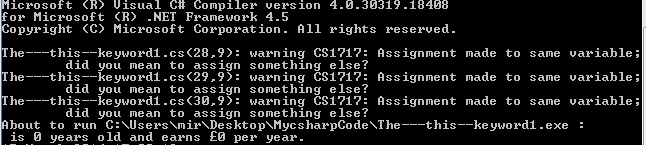Close

# c# – The “this” keyword

Earlier we cam across the following:

[csharp]
using System;
using System.Collections.Generic;
using System.Linq;
using System.Text;

class Employee
{
public string Firstname { get; set; }
//Note, the above line is the shorthand way of writing the following:
//
// private string firstname; // here we are defining a property called "firstname"
// public string Firstname
// {
// get{return firstname;}
// set{firstname = value;}
// }
//
// The same could be done for the following two lines

public int Age { get; set; }
public double Salary { get; set; }

public Employee(string Firstname, int age, double Salary)
{

this.Firstname = Firstname;
this.Age = Age;
this.Salary = Salary;
}

}

class Program
{
static void Main()
{
Employee Jenny = new Employee("Jennifer", 22, 25023.50);
Console.WriteLine("{0} is {1} years old and earns £{2} per year.", Jenny.Firstname, Jenny.Age.ToString(), Jenny.Salary.ToString());
}
}
[/csharp]

If you look at the above code, you will notice that the employee class's constructor has input parameters that are called Firstname, Salary, and Age. But these names are also used as the names of the Employee class's properties.

As a result it can get a bit confusing as to what we are referring to, and consequently the above code will result in an error message:One way to fix this is by using changing the names for the properties/input-parameters. However you would often want the names to match as a way to make it easier to see the link between an input parameter, and the property it sets/gets. That's why a better way is by using the "this" keyword as a label the members that are internal to the class (e.g. private members), i.e. we can rewrite the above code to:

[csharp]using System;
using System.Collections.Generic;
using System.Linq;
using System.Text;

class Employee
{
public string Firstname { get; set; }
public int Age { get; set; }
public double Salary { get; set; }

public Employee(string Firstname, int Age, double Salary)
{

this.Firstname = Firstname; // the "this" keyword that the
this.Age = Age; // left hand side of the equation
this.Salary = Salary; // refers to the property.
}

}

class Program
{
static void Main()
{
Employee Jenny = new Employee("Jennifer", 22, 25023.50);
Console.WriteLine("{0} is {1} years old and earns £{2} per year.", Jenny.Firstname, Jenny.Age.ToString(), Jenny.Salary.ToString());
}
}[/csharp]